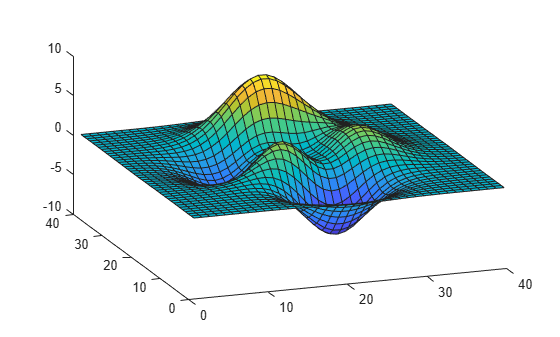## Rotate About an Arbitrary Axis

This example shows how to rotate an object about an arbitrary axis.

### Translate to Origin Before Rotating

Rotations are performed about the origin. Therefore, you need to perform a translation so that the intended axis of rotation is temporarily at the origin. After applying the rotation transform matrix, you then translate the object back to its original position.

### Rotate Surface

This example shows how to rotate a surface about the y-axis.

Create Surface and Transform

Parent the surface to the transform object.

```t = hgtransform; surf(peaks(40),'Parent',t) view(-20,30) axis manual```Create Transform

Set a y-axis rotation matrix to rotate the surface by -15 degrees.

```ry_angle = -15*pi/180; Ry = makehgtform('yrotate',ry_angle); t.Matrix = Ry;```The surface rotated -15 degrees about the y-axis that passes through the origin.

Translate the Surface and Rotate

Now rotate the surface about the y-axis that passes through the point x = 20.

Create two translation matrices, one to translate the surface -20 units in x and another to translate 20 units back. Concatenate the two translation matrices with the rotation matrix in the correct order and set the transform.

```Tx1 = makehgtform('translate',[-20 0 0]); Tx2 = makehgtform('translate',[20 0 0]); t.Matrix = Tx2*Ry*Tx1;```